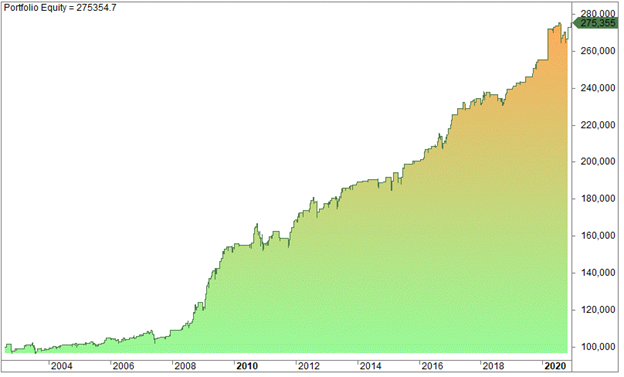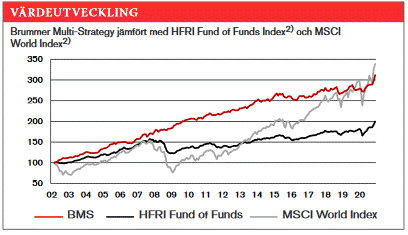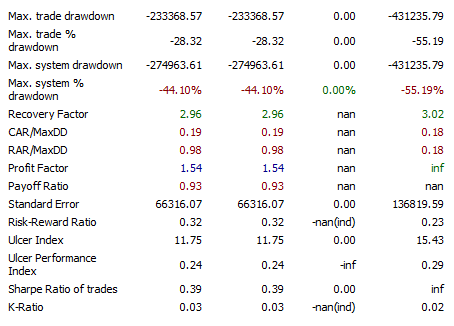Since 2013

• 100% Quantified, data-driven and Backtested
• We always show our results!
• Signals every day via our site or email
• Cancel at any time!

# What Is A Good Profit Factor In Trading?

Last Updated on 11 September, 2023 by Samuelsson

There are many metrics traders use to evaluate a trading strategy. While it’s easy to judge a strategy by the result, such as the CAGR or the annual return, you need the profit factor to analyze the quality of the return and the CAGR. So, what is a good profit factor in trading?

The right profit factor depends on the amount of risk you are willing to endure because there is always a trade-off between assumed risk and payoff. When you want as much profit as possible, be ready to endure more risk. However, a good profit factor in trading has a value of more than 1.75 but not more than 4.

In this article, we will look at the profit factor and explain what it is, as well as how it’s calculated and how you can interpret it. But first, we will explain how to use the equity curve to assess the performance of a trading strategy.

## Understanding an equity curve

The equity curve is a graphical plot of your trading account growth over time. For example, if you want to test a strategy and start with 100 000 trading capital. As you trade, the initial capital grows or depletes over time through the accumulation of gains or losses, as the case may be. If the equity curve slopes upward from the left to the right, the strategy is making money, but if it slopes downwards to the right, the strategy is losing money.

See an example of an equity curve for a trading strategy below:You can see from the chart above that the equity curve started with 100, 000 in 2002 and ended with 275,355 in 2021. The strategy made money but not good enough, considering the time of nearly 20 years.

Look at the chart below. It is a real-life sample of the equity curve of Brummer & Partners Multi-Strategy Fund:The red line represents the performance of the strategy, and the grey line represents the Swedish Total Return Index. While both lines ended up at about the same level, you can notice that the ride has been much less bumpy for the owners of the Brummer funds, which is exactly what investors want — less risk.

One key point in assessing a strategy is that the more smoothly the equity chart rises from the left to the right, the less risky the strategy, as long as it’s not curve-fitted. An equity line that goes like a straight line indicates a good strategy because it doesn’t have big drawdowns. In this case, you may not need many other assessment parameters.

However, in most cases, the equity curve can’t tell you everything you need to know about the performance of the strategy. You would need some quantitative tests to assess the performance of the strategy, and any good trading software has in-built metrics for evaluating strategies. For example, see this excerpt from Amibroker, which shows the standard metrics on every strategy test:There are 13 different metrics, but the Sharpe Ratio is probably the most famous and is widely used among mutual funds and hedge funds. But we like to use the profit factor.

## How do you calculate the profit factor?

The profit factor is the ratio of gross profits to gross losses. You calculate it by dividing the gross profits by the gross losses. For example, assuming a strategy makes 500 in accumulated profits and 250 in losses, the profit factor is 500/250 = 2.

## So, what is a good profit factor in trading?

Our rule of thumb is to see the profit factor higher than 1.75, but not more than 4.

Normally, we want to see higher values than 1.75 because the results are always worse in real-time than in the test. So, there is a need to have a “margin of safety” by selecting strategies with a reasonably high profit factor. A low profit ratio in a test doesn’t offer that margin of safety, and we consider anything from 1.75 and below as not good enough. We prefer to trade strategies with a number above 2.

However, it is not always a case of the higher the better because there could be curve-fitting. Furthermore, too many variables, too few signals, and too short a test period might lead to exceptional results that are unlikely to work on future and unknown data. When you trade such a strategy in the live market, you would be disappointed. Usually, mean-reverting strategies tend to have higher profit factors than trend-following strategies.

## Profit factor and risks

Let’s say you have a strategy that has generated 300 trades over the last decade, and it has a profit factor of 3, which is pretty high. Assuming this strategy uses only two variables, the chances of curve fitting are reduced but not eliminated (it never will be). By tweaking the two variables, you may be able to generate more trades, but you would notice that the profit factor and the max drawdown have gotten worsen. However, the strategy makes more money overall because of the increased number of trades. Thus, there is always a trade-off between assumed risk and payoff. If there is no pain, there is no gain. While you want as much profit as possible, it is necessary to get the profit with the least amount of stress and headache.

With automated systematic trading, you can trade many strategies that can smooth your returns. This is best with a portfolio of quantified strategies. When many strategies are combined, the sum of the strategies might give a high profit factor but most importantly, it reduces risk because of its diversity.

Your diversification can be in different markets, different time frames, and different types of strategies. Your only limit is your creative capacity and not the software to achieve that.

## Final thoughts

The profit factor is a good metric for assessing the performance of a strategy. It is gotten by dividing the gross profits by the gross losses. A good profit factor in trading has a value between 1.75 and 4; so, be skeptical if the value is lower or higher than this range.